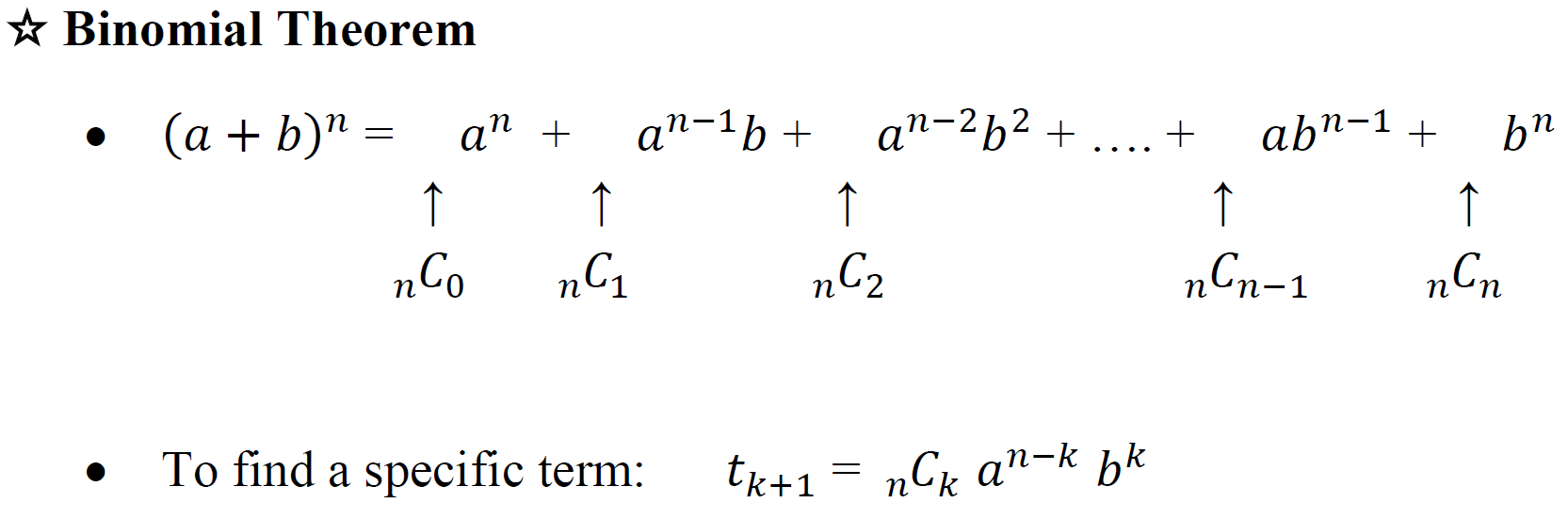Binomial theorem

Binomial theorem

The Binomial Theorem is another method to help us expand binomials in a faster manner. It is particularly useful when we work on binomial expansions that involve binomials raised to high powers.

Lessons• 1.
Expand ${\left( {a + b} \right)^4}$, using:
a)
Pascal's Triangle

b)
Binomial Theorem

• 2.
Expand:
a)
${\left( {5x + 2} \right)^3}$

b)
${\left( {2x - 3y} \right)^4}$

• 3.
In the expansion of ${\left( {\frac{1}{{7{x^2}}} - {x^3}} \right)^{10}}$ , determine:
a)
the 4th term

b)
the middle term

c)
the constant term

• 4.
In the expansion of ${\left( {3 - 2x} \right)^8}$ , determine the coefficient of the term containing ${X^5}$.